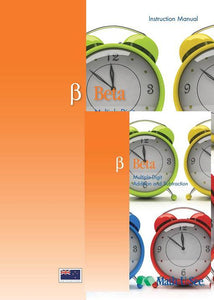or 6 weekly interest-free payments from \$12.50 withwhat's this?Demme Learning

# Math.U.See Beta

Regular price \$65.00 \$40.63 Unit price per
Tax included. Shipping calculated at checkout.

## Math.U.See Beta

#### Addition and subtraction for single-digit numbers and other topics.

Beta builds on the foundation in Alpha by applying students’ mastery of single-digit addition and subtraction to multiple-digit addition and subtraction. One of the stepping stones between these two levels is the concept of place value, which is an important milestone for truly grasping any multiple-digit operation.

Major Concepts and Skills Include:

• Understanding place value and using it to add or subtract
• Fluently adding any combination of whole numbers
• Solving for an unknown addend
• Fluently subtracting any combination of whole numbers
• Solving abstract and real-world problems involving addition and subtraction

• Telling and writing time by hours and minutes
• Understanding, adding, and subtracting NZ currency
• Measuring and estimating length with inches, feet, centimeters, and meters
• Comparing numbers and lengths
• Expressing differences between numbers as inequalities
• Finding the perimeter of any polygon
• Representing and interpreting data in plots and graphs

Beta Instruction Pack includes: Instruction Manual & DVD

Beta Student pack includes: Student Workbook & Test & Activity booklet.

Grab an extra Student Pack for an additional Student.

For a complete set to get started with Math.U.See you will need to purchase the Integer Block set (a one of purchase for use with ALL levels of Math.U.See), the Beta instruction pack & Beta Student Pack